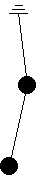Khinchin’s book on statistical mechanics (ISBN=0486601471) introduced me to Hamiltonians. The simple idea there is that H is merely the total energy of the system where H is taken to be some function of the dynamic coordinates pi and qi for 1≤i≤s. This idea is evidently in stark contrast to the historical origin of Hamiltonians which is by way of Lagrangians, in terms of which Hamiltonians are normally introduced.

We require:
 ṗi = ∂H/∂qi q̇i = −∂H/∂pi
(1)
These two equations provide simple means to compute the evolution of pi and qi as time passes. So far we merely have a way to compute the evolution of p’s and q’s if we have a function H of them. H determines the course of the dynamic variables.

I characterize pi and qi as positions and momenta respectively for the following but this formalism can be extended to many other physics problems by taking pi and qi to be other physical quantities so long as the two equations above hold. (See conjugate coordinates.)

### simple oscillator

We consider a simple oscillator first. A mass=m has one degree of freedom x and a spring imposes a force = −hx. Kinetic energy = mx'2/2. When the mass is displaced to x the work against the spring (potential energy) is hx2/2. Using our conventional notions of energy and taking p1 = x/√h; q1 = x'/√m
we propose the following Hamiltonian:
H = ((K.E.) + (P.E.)) = ((mx'2/2) + (hx2/2))/2 = (hp12 + mq12)/2.

Note that if H is a serviceable Hamiltonion then so is bH where b is a constant; H and bH predict the same evolution of the dynamic variables but 2H evolves twice as fast as H.

We try ṗ1 = ∂H/∂q1 and q̇1 = −∂H/∂p1 with our proposed H. ṗ1 = ∂H/∂q1 = mq1 and q̇1 = −∂H/∂p1 = −hp1. These simple differential equations yield:
(p1, q1) = (a sin(√(h/m)t + b), a cos(√(h/m)t + b))
for some constants a and b.

Presumably the reader is not yet impressed of the magic of the Hamiltonian; but wait:

### compound pendulum

Lets take a compound pendulum (toobetter graphics):
 Two masses, one beneath the other. Inelastic links hinged at the top and at the middle mass each mass = 1 each link length = 1 g = 1.The angle of the upper link relative to the vertical is θ1 and of the lower link θ2. pi = θi.

We will need the velocity of the lower weight to compute its kinteic energy. The velocity of the upper weight is d(sin2 θ1,)/dt

H = (K.E.) + (P.E.) = (−2 cos(θ1) − cos(θ2)) + (1/2 (θ12)